# Selection Operations¶

Chapters 4.1 - 4.7

Today, we will focus more attention on the Selection Structure, usually seen in the form of an if-then-else statement: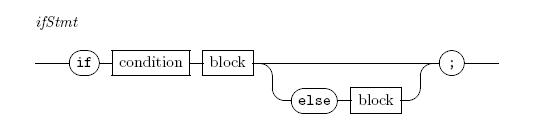We have seen how this statement works, it follows the logical structure we saw in our flowcharts: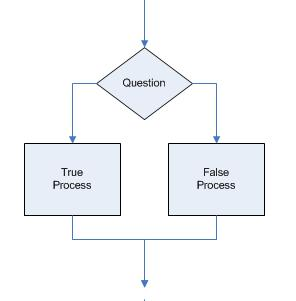Finding ways to use this structure is a fairly simple process - any time you need to ask a question, you will end up using this structure. The big problem is How do we ask questions?

All questions in programming take the form of Logical Expressions that end up delivering a true or false answer. The question (logical expression) is evaluated as part of a decision block and control of the program follows the path indicated by the answer. We cannot go through both paths!

So how do we form Logical Expressions?

## Logical Operators¶

There are a number of obvious and a few not-so-obvious operators used to form our questions. Here they are:

• < less than
• <= Less than or equal
• > greater than
• >= greater than or equal
• == equal to
• != Not equal to

Here are the diagrams we need to finish off our if-then-else statement rules: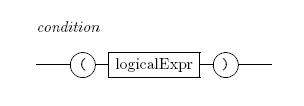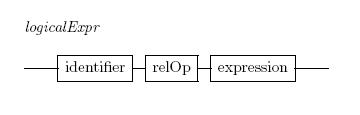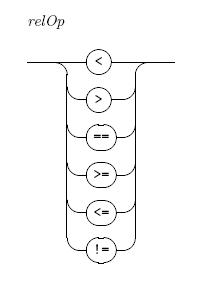We call these operators Relational Operators because they evaluate two things to see what relationship they have to each other.

These operators are used between two operands which is just a fancy name for some value or variable on either side of the operator. What causes confusion when you first start learning about these logical expressions is that those operands on both sides of the operator can be the familiar numerical expressions we have used earlier. Phew!! Let’s work on that part later. For now, we will keep the operands simple.

## Typical Questions¶

if (counter == 3){
cout << "We hit 3!" << endl;
}


The logical expression compares the two values on either side of the operator, then - based on the operator – decides if the answer is true or false. In the case of the selection statement above, the current value stored in the variable counter is compared to the value 3 and if they are equal at that moment, the expression evaluates to true, otherwise it evaluates to false.

### Using Variables in expressions¶

We have shown how to set up variables in previous examples. You must declare them, and tell the system what kind of data you plan to place in them. When the system sees a variable in an expression, it simply looks up the value stored in that variable at that moment and uses that value in the calculations it is doing. In our simple numerical expressions, the system was performing math, in our logical expressions it is performing comparisons.

## More Complex Logical Expressions¶

Suppose you want to know if a value falls between two other values.

For instance, suppose I want to assign a letter grade based on a test score. How would I go about doing that?

### Nested If Statements¶

Well, based on what we know at the moment, we could use nested if-then-else statements:

int score = 92;

if (score >= 90) {
cout << "Your grade was 'A'" << endl;
} else {
if(score >= 80) {
cout << "Your grade was 'B'" << endl;
}
}


You can see how to complete this. When you set up complicated questions like this, you need to think through all the possible cases to make sure your questions will work the way you expect. In ordering the questions as we have above, we start by looking for grades greater than or equal to 90. Then (if the grade is below 90), we look for those above or equal to 80. This will catch all grades between 80 and 89 (see why?). We know the value is not 90 or above because that value would have already been caught by the first part of this question.

If we want to ask more complex questions, we need to introduce two more logical operators:

• && AND
• || OR

These operators allow us to combine smaller logical expressions into a more complicated question. The operands on either side of these operators must evaluate to true or false values. The result of combining the se values with the AND or OR operators can be seen in a simple Truth Table form:

Here is the table for the AND operator (A && B):

A B Result
true true true
true false false
false true false
false false false

We can see that we get a true result only if both A AND B are both true - so it kind of makes sense!

Here is the table for the OR operator (A OR B)

A B Result
true true true
true false true
false true true
false false false

Here we get a true value if Either A or B is true - again it kind of makes sense!

#### Combining Questions¶

We can use these operators to ask more complex questions.

Is this value between one value and another value?

• if (value >= low && value <= high) …

Notice that we do NOT just write something like this:

• WRONG: if (value >= low && <= high) …

That would generate a syntax error. Do you see why - we have two operators in a row, and that is not allowed. So, we need to combine two simple logical expressions with the AND operator to get the result we are after.

#### More on nesting selections¶

Selection statements can get quite involved. You can use selections inside other selections to ask complex questions. The use of nested if statements is not a problem, except that if you use correct style, you end up indenting your code way over to the right, and that does not really help with the readability. Lets look at the nesting concept a bit, and see what is really happening:

Here are two simple if statements in a sequence:

if(grade >= 90 && grade <= 100) {
cout << "Grade is 'A'" << endl;
}

if(grade >= 80 && grade < 90) {
cout << Grade is 'B'" << endl;
}


Is there anything wrong with this?

Not really, the code will work as expected. However, the entire second logical expression will be evaluated by the system even if the first logical expression evaluates to true! We are wasting time, and could mess things up if we get our logical expressions confused.

The solution to these problems is to nest the statements:

if(grade >= 90 && grade <= 100) {
cout << "Grade is 'A'" << endl;
} else {
if(grade >= 80) {
cout << Grade is 'B'" << endl;
}
}


Now you see the inner if statement indenting a bit. In this example, the second logical expression will not even be evaluated if the first expression results in a true - so we are making the program more efficient. Notice that I do not even need to check if the grade is less than 90 since all grades of 90 or better were captured by the first expression.

We can solve the style problem by making use on a new fact about this expression.

So far, every time we have written an if statement, we have used curly braces around the entire true statement set and false statement set. However, the rules for the language let us omit those braces if we only want one statement in that part! So, we can rewrite the above example this way:

if(grade >= 90 && grade <= 100)
cout << "Grade is 'A'" << endl;
else
cout << Grade is 'B'" << endl;


We are still indenting, but we have gotten rid of a bunch of braces. Now, change the style a bit and we have a better solution:

if(grade >= 90 && grade <= 100)
cout << "Grade is 'A'" << endl;
else if(grade >= 80)
cout << Grade is 'B'" << endl;


Now, we have eliminated the indenting, and we can add as many sections as we like. We are still free to have more than one thing happen in each section, but we need to add in the braces if we do:

if(grade >= 90 && grade <= 100)
cout << "Grade is 'A'" << endl;
else if(grade >= 80) {
cout << " Score was " << grade << endl;
cout << Grade is 'B'" << endl;
}


Careful use of this kind of style will make the code easier to read (and write!)

## Building a useful program¶

Take a deep breath, we are going to dive into C++ programming pretty deeply in what follows. Read through this material first. I will talk you through a complete program in pieces. We will build some code, then test it out to see what happens. I will try to explain the thinking going on during this process. You should fire up Dev-C++ and try the code yourself. Nothing beats experimenting with this new thing called programming. As you gain confidence in what you are doing, feel free to cut and paste code fragments and build your own version of the examples.

Let’s try to build a useful program. We want to see if we really are getting a good deal when we buy a car and get “Money Back”, or a good loan rate. (I want both!)

Here is the problem we will explore here:

### Car Loan Decisions¶

Suppose you have picked out a car and arrived at a selling price. The salesman offers you “Cash Back” if you buy the car at one loan rate, or no cash but a better loan rate. Which way should you go.

We want to play with the numbers a bit, so we want to allow the user to enter several sets of data to compare the various situations.

#### Breaking it down¶

Where do we start?

First, let’s identify the information we need:

• Car selling price
• Amount of cash back offered
• Loan interest rate and months
• No cash loan interest rate and months.
• We also need a formula to see how much the car will cost us per month.

I Googled this problem and came up with this link:

payment formula

And, here is the formula - it is a bit complicated and involves a magic operation we will learn about soon - the Power Function:

Let: P be the amount borrowed
N = the number of years for the loan
I = the interest rate (as a fraction - 11% = 0.11)

M is the monthly payment

M = P * I / ( 12 * (1 - pow(1 + (I/12),(-N * 12))))


As an example, suppose we borrow $12000 for 4 years at 11%. The monthly payment would be: • 12000 * 0.11 /(12 * (1 - pow(1 + (0.11/12),(-4*12)))) Here, the pow(a,b) function gives the result of raising a to the b power. In our sample problem, both a and b are expressions. We will make this clearer once we talk about functions in the course - for now, trust me, it will work as we need it to. All of this messy formula is something you should have worked through in your math class. And financial formulas are as messy as any! We just have to trust the formula, and let the computer do it’s thing. It sure beats running the formula through your calculator! If we do it right, the sample loan gives a result of$310.15. Let’s code this part up and see if we can get it to work.

#### Floating point data¶

For this problem, we need to use floating point data. Remember that this kind of data is just a number encoded in such a way that it can have a fractional part. For our calculations here, we will use a high precision floating point number called double. This is another of the built-in data types that C++ knows about - the more bits used to encode the numbers, the better the accuracy of the calculations. Here is a code fragment to calculate the monthly payment:

#include <cmath>

...

double P = 12000.00;
double I = 0.11;
double N = 4.0;
double M;

M = P * I / (12 * (1 - pow(1 + (I/12),(-N * 12))));

cout << "Monthly payment = " << M << endl;


That #include line makes it possible for us to use those magic math functions we need for this problem. We have seen this before!

Note

All C++ programs start off with a basic structure you have already seen - the empty code produced by Dev-C++ when you start a new project. To make this fragment work, you start a new project and add this fragment to the code. The #include part goes after the existing #include <iostream> line, and the rest of the fragment goes inside the curly brackets that make up the main part of the code. The compiler will tell you when you get it right! (Do not include the “…” line.)

Does it work? Let’s see:

Monthly payment = 310.146
Press any key to continue . . .


For now, we will not worry about the actual number being a weird fraction.

It works!

What we just did was a research project to make sure we had all the information we needed to solve this problem, and we knew what to do with that information. The website showed us a complex formula, that we needed to test to see if we could get good results. So, we coded up a little test program and tried it out - working with it until we got the right answer to a test problem found on the website. With that done, we feel confident that we can proceed!

#### Getting started - setting up the loop¶

Since we want the user to be able to try a bunch of options, we will need to set up a loop for the program. We will ask the user for some information, and use something called a sentinel value to control bailing out of the loop:

Here is what it looks like as a flow chart: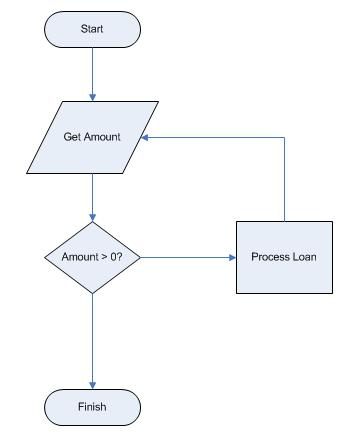Do you see how we use a special value to bail out of the loop. You cannot borrow negative money, so we let the user enter a negative loan amount to get out of the loop.

We can translate this diagram into C++ as follows:

double P = 1.0;

while(p > 0) {
cout << "Enter the loan amount:";
cin >> P;
cout << "process the loan for " << P << endl;
}


Do you see a problem with this translation? The code is not exactly like the diagram. I cheated and started off by initializing the value of the variable P in the declaration and used this starting value to make sure I start the loop. We get the real first value from the user once we get things going. This is one way to start the loop, but maybe not the best. In any case, once we get into the actual loop, it works like we want.

This fragment shows how to get information from the user. We have been using a similar statement to display things on the screen using that cout name and the double ** << ** brackets. Here, we use the cin name and the double ** >> ** brackets to get user data from the keyboard into the variable named P in this example. (You might ask if the name P is a good one - not really, but the name does match that formula we found earlier!) Assuming the user does what we ask on the prompt we write out before the input line, whatever the user types will be loaded into our variable for the program to use from that point.

This tiny fragment is enough to test our program logic and see if it works:

Enter a loan amount:10000
Processing loan for 10000
Enter a loan amount:20000
Processing loan for 20000
Enter a loan amount:30000
Processing loan for 30000
Enter a loan amount:-1
Processing loan for -1
Press any key to continue . . .


Well, it works, but I probably do not want to process the loan for the negative amount. Could I rework this code to make it better? Let’s try:

First, notice that we want the user to enter a loan amount even to stop the program. Why don’t we get the first value outside the loop, then again inside the loop? Like this:

cout << "Enter a loan amount:";
cin >> P;
while (P > 0) {
cout << "Processing loan for " << P <<endl;
cout << "Enter a loan amount:";
cin >> P;
}


I had to code up the prompt twice, but the program works better!

Which gives this:

Enter a loan amount:100
Processing loan for 100
Enter a loan amount:200
Processing loan for 200
Enter a loan amount:-1
Press any key to continue . . .


Wait a minute, look back at the flowchart I constructed earlier. In fact, that chart matches the code we just created better than the first try. Hmmm, maybe I was smarter than I thought - or I just was not careful when I translated my diagram into code!

#### Adding in the Monthly Payment calculation¶

In order to move forward, we now need more data from the user. Specifically, we need the number of years (N) and the interest rate (I). Let’s add that in:

cout << "Enter a loan amount:";
cin >> P;
while (P > 0) {
cout << "Enter the number of years for this loan:";
cin >> N;

cout << "Enter the interest rate as a fraction":;
cin >> I;

cout << "Processing loan for " << P <<endl;
M = P * I / (12 * (1 - pow(1 + (I/12),(-N * 12))));
cout << "Monthly payment = " << M << endl;
cout << "Enter a loan amount:";

cin >> P;
}


Now, that pow function is a bit messy. Look back at how it was defined - pow(a,b). Where we see the names a and b, we are actually putting in expressions from our formula to figure out the right values to send into the function. Don’t worry about what is happening here, we are doing the same things you could do with your calculator, figuring out values and pushing function keys to figure out things!

Running our test case shows that this works:

Enter a loan amount:12000
Enter the number of years for this loan:4
Enter the interest rate as a fraction:0.11
Processing loan for 12000
Monthly payment = 310.146
Enter a loan amount:


#### Computing total cost of the loan¶

Next, let’s see how much all the payments add up to. This is an easy step, since all we need to do is a simple multiplication:

double total_cost;
...

total_cost = N * 12 * M;
cout << "This loan will cost you " << total_cost << endl;
cout << "   (" << total_cost - P << " in interest!)" << endl;


Please be careful here, I have had students type in the … stuff above and get confused when the compiler choked! That line means other stuff goes here, and you should be able to look back and figure what that stuff is.

If not, send me an email and I will help clear it up for you.

Enter a loan amount:12000
Enter the number of years for this loan:4
Enter the interest rate as a fraction:0.11
Processing loan for 12000
Monthly payment = 310.146
This loan will cost you 14887
(2887.02 in interest!)
Enter a loan amount:


Well, the numbers do not look like money, but the answers are usable at this point.

#### Adding in cash back¶

At this point, we need to think about where we are trying to go, and modify our code to suit. Let’s add some comments into our code as to help keep track of what we want to do. A comment begins with the two characters // and extends to the end of that line:

// get the price of the car we are considering
cout << "Enter a loan amount:";
cin >> P;
while(P > 0) {
// see how long we are going to pay for this car
cout << "Enter the number of years for this loan:";
cin >> N;

// get the no cash data
cout << "Enter the interest rate as a fraction:";
cin >> I;

// process the payment and total cost for this option
cout << "Processing loan for " << P <<endl;
M = P * I / (12 * (1 - pow(1 + (I/12),(-N * 12))));
cout << "Monthly payment = " << M << endl;
total_cost = N * 12 * M;
cout << "This loan will cost you " << total_cost << endl;
cout << "   (" << total_cost - P << " in interest!)" << endl;

// get the cash back option
// read the amount of cash back ( we will apply it to the loan)
// get the new interest rate
// process the cash back option

cout << "Enter a loan amount:";
cin >> P;
}


So far, we have developed the program to the point where we can figure out how much the car will cost for a basic loan. What remains is to add in the cash back option. In this exercise, we will use the cash back to buy down the loan. That means that instead of a new big-screen TV, we will apply the cash back to the car price and finance less money (that is what you would do - right?)

So, how do we proceed?

First, we need to get the data from the sales-droid. How much cash are we going to get back, and what will the interest be on the loan?

Here is some sample data for you to work with:

• Price for car: 20000
• Loan time: 5 years
• Cash back interest rate: 5%
• Cash back: 1500
• No cash interest rate: 1%
• Cash back payments: $349 • No cash back payment:$342
• Total price with cash back: $20947 • Total price no cash:$ 20512

Our work involves replacing the comments with actual code.

Here is code to get the additional data from the user:

double cash_back, cash_back_interest;
...

cout << "How much cash back will you get?:";
cin >> cash_back;
cout << "What is the normal interest rate?:";
cin >> cash_back_interest;


Now, we can finish off by calculating the new payment and total cost:

double M2;
...
P = P - cash_back;
I = cash_back_interest;
M = P * I / (12 * (1 - pow(1 + (I/12),(-N * 12))));
total_cost2 = M * 12 * N;
cout << "Your cash back payment will be: " << M << endl;
cout << "This loan will cost you " << total_cost2 << endl;
cout << "   (" << total_cost2 - P << " in interest!)" << endl;


Notice that we lowered the amount we need to finance by the amount of the cash back. In order to reuse a complicated line of code, I also copied the cash back interest rate into the I variable so the original formula would still work.

All that remains is to see which way is smarter - cash or no cash!

if (total_cost > total_cost2) {
cout << "Skip the cash!" << endl;
} else {
cout << "Take the cash!" << endl;
}


And we have a useful tool!

But it is ugly!

As programs go, this one is OK, but could use some cleaning up. Here is the total program as we now have it:

#include <cstdlib>
#include <iostream>
#include <cmath>

using namespace std;

int main(int argc, char *argv[])
{
double P;
double I;
double N;
double M;
double total_cost, total_cost2;
double cash_back, cash_back_interest;

// get the loop started
cout << "Enter a loan amount:";
cin >> P;
while(P > 0) {

// get the length of the loan in years
cout << "Enter the number of years for this loan:";
cin >> N;

// now, get the interest rate as a fraction (11% is 0.11)
cout << "Enter the interest rate as a fraction:";
cin >> I;

// process the basic loan to get the payments
cout << "Processing loan for " << P <<endl;
M = P * I / (12 * (1 - pow(1 + (I/12),(-N * 12))));
cout << "Monthly payment = " << M << endl;

// now compute the total cose
total_cost = N * 12 * M;
cout << "This loan will cost you " << total_cost << endl;

// see how much cash back we are being offered
cout << "   (" << total_cost - P << " in interest!)" << endl;
cout << "How much cash back will you get?:";
cin >> cash_back;

// see what the cash back interest rate is
cout << "What is the new cash back interest rate?:";
cin >> cash_back_interest;
P = P - cash_back;
I = cash_back_interest;

// figure out the new payment and total cost
M = P * I / (12 * (1 - pow(1 + (I/12),(-N * 12))));
total_cost2 = M * 12 * N;
cout << "Your cash back payment will be: " << M << endl;
cout << "This loan will cost you " << total_cost2 << endl;
cout << "   (" << total_cost2 - P << " in interest!)" << endl;

// now figure out the best deal
if (total_cost2 > total_cost) {
cout << "Skip the cash!" << endl;
} else {
cout << "Take the cash!" << endl;
}
cout << "Enter a loan amount:";
cin >> P;
}
//cout << "Monthly payment = " << M << endl;
system("PAUSE");
return EXIT_SUCCESS;
}


Now, we talked our way through this code as we wrote it all down. Actually, if you look at the comments, you see that we are capturing our thinking (talking) as comments in the code to make it clearer what is going on.

Are we done yet? Not quite. One problem remains. The quick and dirty names I chose for the variables are not that clear. While short names are nice when typing stuff in, they can be a pain over the life of the program since they may not be that clear. Let’s rename a few things so the names we are using more properly indicate what is going on, and modify the output to make it neater:

#include <cstdlib>
#include <iostream>
#include <cmath>

using namespace std;

int main(int argc, char *argv[])
{
// Define the variables we need here
double car_price;
double loan_years;
double no_cash_interest, cash_back_interest;
double cash_back;
double monthly_payment;
double no_cash_cost, cash_back_cost;

// Get the first loan amount to start the loop
cout << "Enter a loan amount (negative to quit):";
cin >> car_price;

// repeat the analysis for each loan the user is considering
while(car_price > 0) {
cout << "Processing loan for " << car_price <<endl;

// how long will the loan be for?
cout << "Enter the number of years for this loan:";
cin >> loan_years;

// Part 1 - no cash back option (need an interest rate)
cout << "Enter the interest rate as a fraction:";
cin >> no_cash_interest;

// do the no cash back calculations
monthly_payment = car_price * no_cash_interest /
(12 * (1 - pow(1 + (no_cash_interest/12),(-loan_years * 12))));
no_cash_cost = loan_years * 12 * monthly_payment;

// show the results
cout << "No cash back option results:" << endl;
cout << "    Monthly payment = " << monthly_payment << endl;
cout << "    This loan will cost you " << no_cash_cost;
cout << " (" << no_cash_cost - car_price << " in interest!)" << endl;

// Part 2 - Cash back option (Need interest and cash amount)
cout << endl << "How much cash back will you get?:";
cin >> cash_back;
cout << "What is the cash back interest rate?:";
cin >> cash_back_interest;

// do the cash back calculations
car_price = car_price - cash_back;
monthly_payment = car_price * cash_back_interest /
(12 * (1 - pow(1 + (cash_back_interest/12),(-loan_years * 12))));
cash_back_cost = monthly_payment * 12 * loan_years;

// Show the cash back results
cout << "Cash back option results:" << endl;
cout << "    Your cash back payment will be: " << monthly_payment << endl;
cout << "    This loan will cost you " << cash_back_cost;
cout << "   (" << cash_back_cost - car_price << " in interest!)" << endl;

// show which option is best
cout << "My recommendation: "
if (cash_back_cost > no_cash_cost) {
cout << "Skip the cash!" << endl;
} else {
cout << "Take the cash!" << endl;
}
cout << "Enter a loan amount (negative to quit):";
cin >> car_price;
}

// pause the program on exit
system("PAUSE");
return EXIT_SUCCESS;
}


Notice that I have sprinkled a few extra endl items into my output to space things out a bit, and I have indented some of the output - all to make the program more usable.

Here is the final output - neater now!

Enter a loan amount (negative to quit):20000
Processing loan for 20000
Enter the number of years for this loan:5
Enter the interest rate as a fraction:0.01

No cash back option results:
Monthly payment = 341.875
This loan will cost you 20512.5 (512.497 in interest!)

How much cash back will you get?:1500
What is the cash back interest rate?:0.05

Cash back option results:
Your cash back payment will be: 349.118
This loan will cost you 20947.1   (2447.07 in interest!)
My recommendation: Skip the cash!
Enter a loan amount (negative to quit):


That should do it!